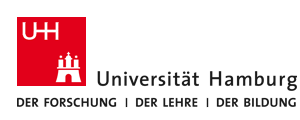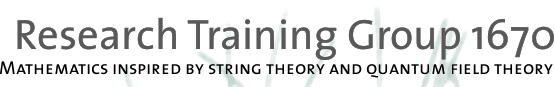# Core Research Area D: Differential geometry and supersymmetry

For many years, there has been a fruitful interplay between supersymmetry, string theory and differential geometry [C]. In this Core Research Area (CRA) we continue to explore the the geometry of deformation spaces of geometrical structures related to the scalar field spaces of supergravity and string theory. Of particular interest are four-dimensional N = 2 supergravities whose scalar field space locally is a product of a special Kähler and a quaternionic Kähler manifold. The special Kähler manifold arising in the low energy effective action of string theories is reasonably well understood, while comparatively little is known about the quaternionic Kähler component as it generically receives perturbative and non-perturbative quantum corrections. It arises in the hypermultiplet sector of N=2 type II and heterotic compactification and thus is related to properties of K3 and/or Calabi-Yau threefolds.

The following projects are current and future topics in the CRA:

• Study of the hypermultiplet metric in string compactifications arising from Calabi-Yau manifolds with vanishing Euler number. Building on earlier work [CLST] [DLMST] [HLS] [LST10] it was shown in [KMT] that they also admit a non-integrable SU(2)-structure corresponding to a phase of spontaneously broken N = 4 -> N = 2 supergravity which in turn constrains the possible quantum corrections.
• Extending the study of supersymmetric AdS_4 backgrounds, their moduli spaces and their holographically dual superconformal field theory along the lines of [DLMTW] [LST12] [LT] to other dimensions and to consistent truncations of 10/11-dimensional supergravity of the form AdS_d x Sasaki-Einstein spaces.
• Dimensional reduction of supergravity theories and perturbative quantum corrections are methods inspired by string theory which, based on research in our group [CHM] [CDL] [CNS] [D], can be effectively used to construct many new complete quaternionic Kähler manifolds of negative scalar curvature. We are systematically studying the geometric properties of these constructions.
• It would be nice to have a mathematical description of non-perturbative quantum corrections to quaternionic Kähler metrics, such as the metric on the hypermultiplet sector of type II string theory compactified on a Calabi-Yau three-fold. In particular, it is not known in which situations non-perturbative corrections lead to complete metrics. Perturbative corrections of the hypermultiplet moduli space can be described using a one-parameter deformation of a c-map metric induced by the HK/QK-correspondence [APP] [ACM] [ACDM]. The method can be also applied in the case of other interesting moduli spaces such as the Hitchin system.